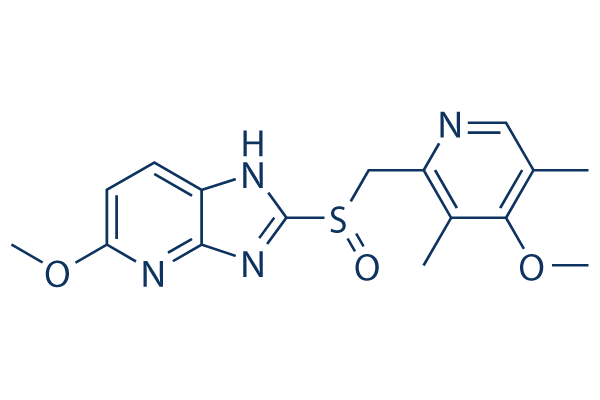# Tenatoprazole

For research use only.

Catalog No.S4212 Synonyms: TU-199

1 publicationCAS No. 113712-98-4

Tenatoprazole (TU-199) is a prodrug of the proton pump inhibitor (PPI) class, which inhibits proton transport with IC50 of 3.2 μM. Tenatoprazole labels only the gastric H(+),K(+)-ATPase alpha-subunit, approximately 2.6 nM/mg of tenatoprazole is bound to the H(+),K(+)-ATPase.

### Biological Activity

Description Tenatoprazole (TU-199) is a prodrug of the proton pump inhibitor (PPI) class, which inhibits proton transport with IC50 of 3.2 μM. Tenatoprazole labels only the gastric H(+),K(+)-ATPase alpha-subunit, approximately 2.6 nM/mg of tenatoprazole is bound to the H(+),K(+)-ATPase.
Targets
 Proton pump () H(+),K(+)-ATPase (Cell-free assay) 3.2 μM
In vitro

Tenatoprazole labeled only the gastric H+,K+-ATPase alpha-subunit as has been found for other PPIs, approximately 2.6 nmol/mg of tenatoprazole is bound to the H+,K+-ATPase. The two enantiomers (R)- or (S)-tenatoprazole give the same stoichiometry of binding with 88% inhibition. Tenatoprazole labels only the peptide containing fifth and sixth transmembrane segments, these contain two cysteines, cysteine 813 in the luminal vestibule and cysteine 822 in the sixth transmembrane domain. 

In vivo Tenatoprazole provides slow activation in vivo, which is predicted by its chemical activation rate in fasting rats. Tenatoprazole inhibits about 20–30% of enzyme activity even though acid secretion in fasting rats. (S)-tenatoprazole sodium salt hydrate provides a higher Cmax of 183 ng/mL, Tmax of 1.3 hours and AUC of 822 ng*h/mL in dog. 

### Solubility (25°C)

 In vitro DMSO 46 mg/mL (132.79 mM) Ethanol 14 mg/mL warmed (40.41 mM) Water Insoluble

* Please note that Selleck tests the solubility of all compounds in-house, and the actual solubility may differ slightly from published values. This is normal and is due to slight batch-to-batch variations.

Molecular Weight 346.4 C16H18N4O3S 113712-98-4 3 years-20°C powder 2 years-80°C in solvent TU-199 CC1=CN=C(C(=C1OC)C)CS(=O)C2=NC3=C(N2)C=CC(=N3)OC

### In vivo Formulation Calculator (Clear solution)

 Step 1: Enter information below (Recommended: An additional animal making an allowance for loss during the experiment) Dosage mg/kgAverage weight of animals gDosing volume per animal ulNumber of animals Step 2: Enter the in vivo formulation (This is only the calculator, not formulation. Please contact us first if there is no in vivo formulation at the solubility Section.) % DMSO+ % PEG300 Corn oil + % Tween 80+ % ddH2O CalculateReset

### Bio Calculators

Molarity Calculator

## Molarity Calculator

Calculate the mass, volume or concentration required for a solution. The Selleck molarity calculator is based on the following equation:

### Mass (mg) = Concentration (mM) × Volume (mL) × Molecular Weight (g/mol)

• Mass
Concentration
Volume
Molecular Weight

*When preparing stock solutions, please always use the batch-specific molecular weight of the product found on the via label and SDS / COA (available on product pages).

Dilution Calculator

## Dilution Calculator

Calculate the dilution required to prepare a stock solution. The Selleck dilution calculator is based on the following equation:

### Concentration (start) x Volume (start) = Concentration (final) x Volume (final)

This equation is commonly abbreviated as: C1V1 = C2V2 ( Input Output )

• C1
V1
C2
V2

* When preparing stock solutions always use the batch-specific molecular weight of the product found on the vial label and SDS / COA (available online).

## The Serial Dilution Calculator Equation

• ### Computed Result

• C1=C0/X C2=C1/X C1: LOG(C1): C2: LOG(C2): C3: LOG(C3): C4: LOG(C4): C5: LOG(C5): C6: LOG(C6): C7: LOG(C7): C8: LOG(C8):
Molecular Weight Calculator

## Molecular Weight Calculator

Enter the chemical formula of a compound to calculate its molar mass and elemental composition:

### Total Molecular Weight: g/mol

Tip: Chemical formula is case sensitive. C10H16N2O2 c10h16n2o2

#### Instructions to calculate molar mass (molecular weight) of a chemical compound:

To calculate molar mass of a chemical compound, please enter its chemical formula and click 'Calculate'.

#### Definitions of molecular mass, molecular weight, molar mass and molar weight:

Molecular mass (molecular weight) is the mass of one molecule of a substance and is expressed in the unified atomic mass units (u). (1 u is equal to 1/12 the mass of one atom of carbon-12)
Molar mass (molar weight) is the mass of one mole of a substance and is expressed in g/mol.

#### Molarity Calculator

 Mass Concentration Volume Molecular Weight pg ng μg mg g kg = fM pM nM μM mM M × nL μL mL L ×

### Tech Support

Answers to questions you may have can be found in the inhibitor handling instructions. Topics include how to prepare stock solutions, how to store inhibitors, and issues that need special attention for cell-based assays and animal experiments.

Tel: +1-832-582-8158 Ext:3